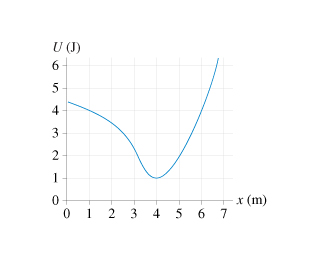# Problem: is the potential-energy diagram for a 20 g particle that is released from rest at x = 1.0 m.What is the particles maximum speed?Where are the turning points of the motion?Will the particle move to the right or to the left?At what position does it have this speed?

⚠️Our tutors found the solution shown to be helpful for the problem you're searching for. We don't have the exact solution yet.

###### Problem Detailsis the potential-energy diagram for a 20 g particle that is released from rest at x = 1.0 m.

What is the particles maximum speed?

Where are the turning points of the motion?

Will the particle move to the right or to the left?

At what position does it have this speed?